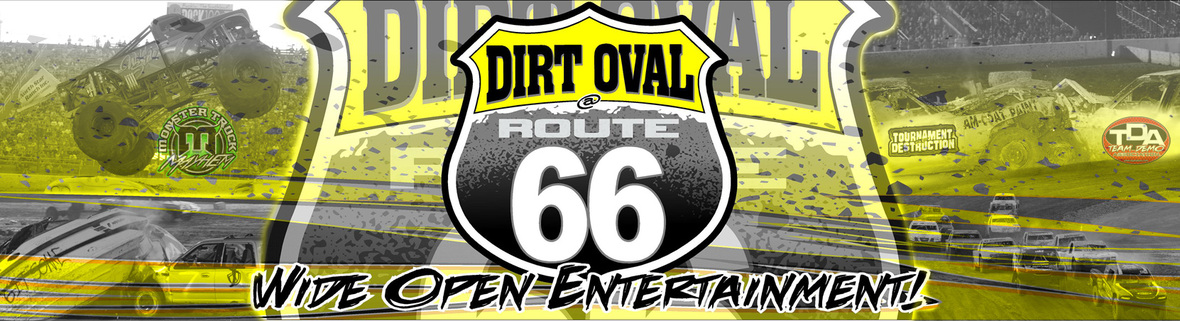Tournament of Destruction Round 4: Next Saturday, August 26th

 table.module-2{width:84.91%;padding:0}table div table+table+table div table{width:84.91%;float:none;margin-left:auto;margin-right:auto;padding:0}table div table+table+table div table a{border:0 none;text-decoration:none}table div table+table+table div table img{width:100%!important;border:0 none;text-decoration:none}table div table+table+table div table td{width:100%;padding:0}/* styles */

'17 Championship up for grabs. Only two events remain!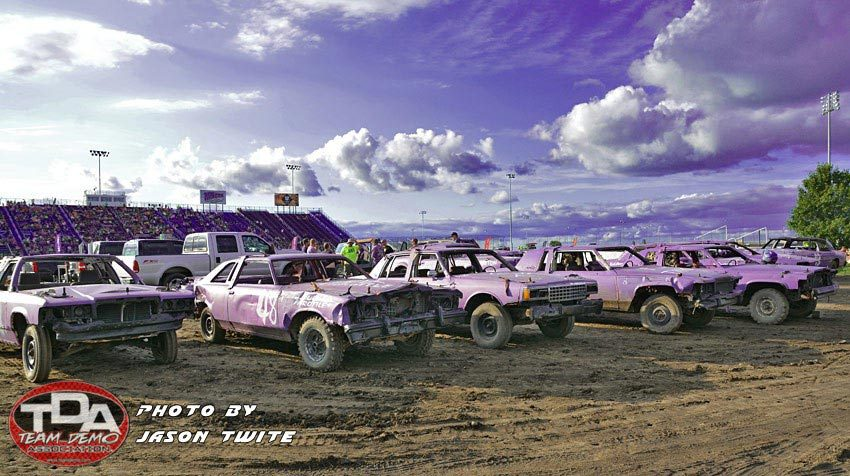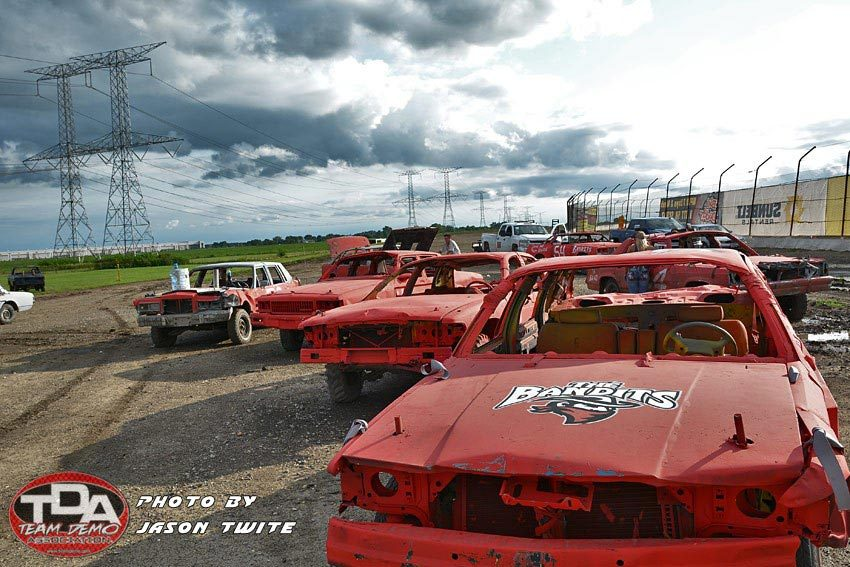/* styles */ Which colors will be flying high at the end of Round 4? Full Throttle and Bandits are two teams yet to raise the trophy in this year's Tournament. Round 1, 2 and 3 produced three different winners; Is a 4th in the fates next Saturday? Its only happened one time in the previous 17 years. That was in 2015 when Orange Crush, Junkyard Dogs, Full Throttle and Mean Green Machine won 4 out of 5 events. Orange Crush picked up a second victory which was pivotal to their 2015 Championship. 3 WAYS TO GET TICKETS: Buy now @ TeamDemo.com Call 815-740-8000 At the Dirt Oval office Zero processing fees with in-person purchase. Kids 2yrs & under are FREE. Call us about group discounts.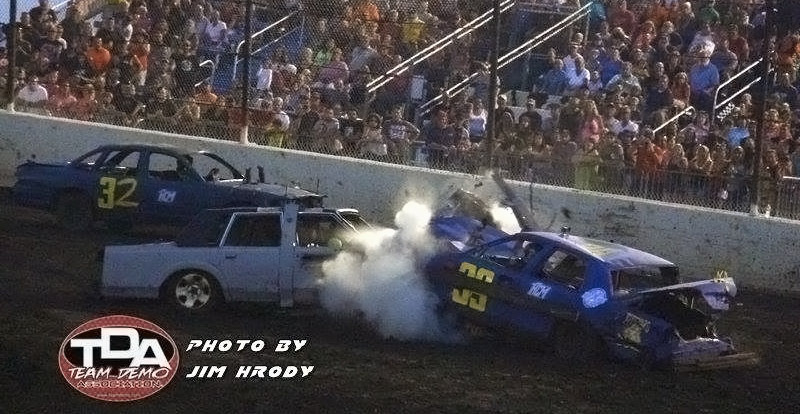/* styles */ War Machine and Junkyard Dogs are also ready to strike! There is zero room for error. Every race is a must win!
 table.module-8{width:75.66%;padding:0}table div table+table+table+table+table+table+table+table+table div table{width:75.66%;float:none;margin-left:auto;margin-right:auto;padding:0}table div table+table+table+table+table+table+table+table+table div table a{border:0 none;text-decoration:none}table div table+table+table+table+table+table+table+table+table div table img{width:100%!important;border:0 none;text-decoration:none}table div table+table+table+table+table+table+table+table+table div table td{width:100%;padding:0}/* styles */

Assemble the Troops and Make Camp for a Weekend at the TrackAwesome activities and interactive learning for all Scouts

Team Demolition Derby's annual Scout Night theme has grown into a full weekend due to increased participation and popularity year after year. Come for everything or only a few activities! Any and all participation is welcome.

View the weekend timeline and register online at DirtOval66.com.

Be prepared for all this and MORE: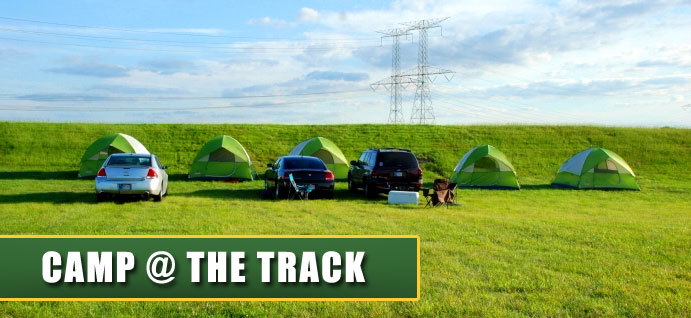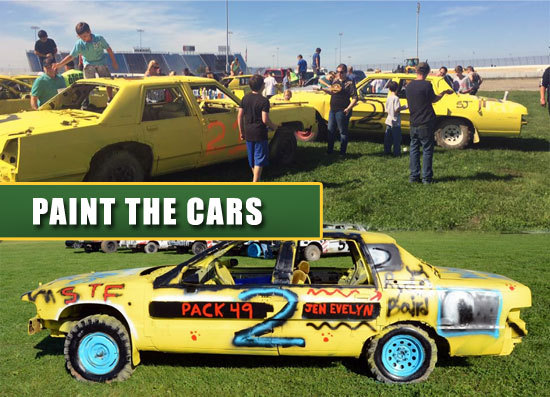/* styles */ Call the Dirt Oval office at 815-740-8000 to register your troop/pack and coordinate group tickets for the Tournament of Destruction Round 4 event on Saturday night, August 26th.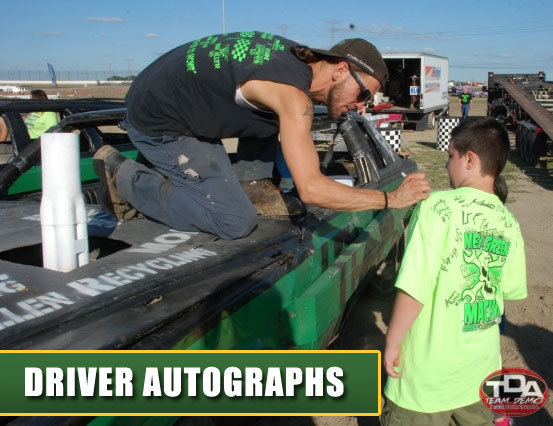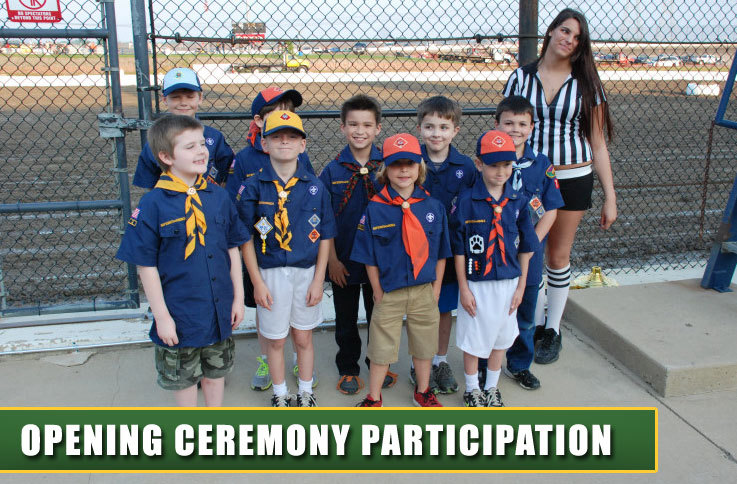/* styles */ Secure your camp site and make plans soon! Contact Samantha@dirtoval66.com with any questions.
 table div table+table+table+table+table+table+table+table+table+table+table+table+table+table+table+table+table+table+table div table td,table.module-18{width:100%;padding:0}table div table+table+table+table+table+table+table+table+table+table+table+table+table+table+table+table+table+table+table div table{width:100%;float:none;margin-left:auto;margin-right:auto;padding:0}table div table+table+table+table+table+table+table+table+table+table+table+table+table+table+table+table+table+table+table div table a{border:0 none;text-decoration:none}table div table+table+table+table+table+table+table+table+table+table+table+table+table+table+table+table+table+table+table div table img{width:100%!important;border:0 none;text-decoration:none}/* styles */

Trucks. Mud. Music. Come Get Dirty!

 table div table+table+table+table+table+table+table+table+table+table+table+table+table+table+table+table+table+table+table+table+table div table td,table.module-20{width:100%;padding:0}table div table+table+table+table+table+table+table+table+table+table+table+table+table+table+table+table+table+table+table+table+table div table{width:100%;float:none;margin-left:auto;margin-right:auto;padding:0}table div table+table+table+table+table+table+table+table+table+table+table+table+table+table+table+table+table+table+table+table+table div table a{border:0 none;text-decoration:none}table div table+table+table+table+table+table+table+table+table+table+table+table+table+table+table+table+table+table+table+table+table div table img{width:100%!important;border:0 none;text-decoration:none}/* styles */

Enjoy Labor Day Weekend in the MUD

Gates open at Noon | Mud flies at 1pm until it dries up!

Bring your truck and bring your Redneck-side to the Inaugural Redneck Jamboree at The Dirt Oval.
Live Music all day | Mega Trucks | Tug-o-Wars | Burnouts
Competition entry details coming soon!

All Access passes are \$20 thru Aug 27th | \$25 day-of.
Contact the Dirt Oval 66 ticket office for your pass!
815-740-8000 | info@dirtoval66.com

A Fall brawl is brewing. Eve of Haunted Destruction - October 7th/* styles */ No one wants to think about the end Summer. Winter is coming, but there is one last smash for the 2017 season! School Bus Race | Extreme Trailer Race | Kids Power Wheels Demo Derby | Full Size, Compact and Lawn Mower Demolition Derby | and who knows what else might be destroyed! There will be chills and plenty of spills! Advanced Tickets are on sale now at DirtOval66.com! Look at just a taste of the carnage to come: 2016 Photo Gallery
 table div table+table+table+table+table+table+table+table+table+table+table+table+table+table+table+table+table+table+table+table+table+table+table+table+table+table div table{width:100%;padding:0}table div table+table+table+table+table+table+table+table+table+table+table+table+table+table+table+table+table+table+table+table+table+table+table+table+table+table div table table{padding:0;float:left!important;width:54.716%!important}table div table+table+table+table+table+table+table+table+table+table+table+table+table+table+table+table+table+table+table+table+table+table+table+table+table+table div table table+table td,table div table+table+table+table+table+table+table+table+table+table+table+table+table+table+table+table+table+table+table+table+table+table+table+table+table+table div table td{padding-left:0;padding-right:0}table div table+table+table+table+table+table+table+table+table+table+table+table+table+table+table+table+table+table+table+table+table+table+table+table+table+table div table table td{padding-left:0;padding-right:20px}table div table+table+table+table+table+table+table+table+table+table+table+table+table+table+table+table+table+table+table+table+table+table+table+table+table+table div table table+table{float:left!important;width:45.284%!important}/* styles */

Outlaws will overrun Dirt Oval 66 in October

 table div table+table+table+table+table+table+table+table+table+table+table+table+table+table+table+table+table+table+table+table+table+table+table+table+table+table+table+table div table td,table.module-27{width:100%;padding:0}table div table+table+table+table+table+table+table+table+table+table+table+table+table+table+table+table+table+table+table+table+table+table+table+table+table+table+table+table div table{width:100%;float:none;margin-left:auto;margin-right:auto;padding:0}table div table+table+table+table+table+table+table+table+table+table+table+table+table+table+table+table+table+table+table+table+table+table+table+table+table+table+table+table div table a{border:0 none;text-decoration:none}table div table+table+table+table+table+table+table+table+table+table+table+table+table+table+table+table+table+table+table+table+table+table+table+table+table+table+table+table div table img{width:100%!important;border:0 none;text-decoration:none}/* styles */

Two Days of 'Wide Open' Dirt Racing | October 13-14

The World of Outlaws Craftsman® Late Models Series roars back into the Dirt Oval @ Route 66 Raceway for their last stop en route to the World Finals in Vegas! Friday, October 13th and Saturday the 14th featuring a full Late Models program plus UMP Modifieds and Street Stock both nights!

Reminder: All tickets from the June 28th FVP Outlaw Clay Classic
are valid for Friday, October 13th!
Contact the Dirt Oval 66 ticket office with questions
815-740-8000 | info@dirtoval66.com

More details coming soon including bonus racing action on Thursday, October 12th! Save \$5 per Adult ticket prior to October 9th. Kids are just \$10 and all seats are general admission.

 table div table+table+table+table+table+table+table+table+table+table+table+table+table+table+table+table+table+table+table+table+table+table+table+table+table+table+table+table+table+table div table{width:100%;padding:0}table div table+table+table+table+table+table+table+table+table+table+table+table+table+table+table+table+table+table+table+table+table+table+table+table+table+table+table+table+table+table div table table{padding:0;float:left!important;width:58.562%!important}table div table+table+table+table+table+table+table+table+table+table+table+table+table+table+table+table+table+table+table+table+table+table+table+table+table+table+table+table+table+table div table td{padding-left:28px;padding-right:28px}table div table+table+table+table+table+table+table+table+table+table+table+table+table+table+table+table+table+table+table+table+table+table+table+table+table+table+table+table+table+table div table table td{padding-left:0;padding-right:20px}table div table+table+table+table+table+table+table+table+table+table+table+table+table+table+table+table+table+table+table+table+table+table+table+table+table+table+table+table+table+table div table table+table{float:left!important;width:41.438%!important}table div table+table+table+table+table+table+table+table+table+table+table+table+table+table+table+table+table+table+table+table+table+table+table+table+table+table+table+table+table+table div table table+table td{padding-left:0;padding-right:0}/* styles */
 table div table+table+table+table+table+table+table+table+table+table+table+table+table+table+table+table+table+table+table+table+table+table+table+table+table+table+table+table+table+table+table div table td,table.module-30{width:100%;padding:0}table div table+table+table+table+table+table+table+table+table+table+table+table+table+table+table+table+table+table+table+table+table+table+table+table+table+table+table+table+table+table+table div table{width:100%;float:none;margin-left:auto;margin-right:auto;padding:0}table div table+table+table+table+table+table+table+table+table+table+table+table+table+table+table+table+table+table+table+table+table+table+table+table+table+table+table+table+table+table+table div table a{border:0 none;text-decoration:none}table div table+table+table+table+table+table+table+table+table+table+table+table+table+table+table+table+table+table+table+table+table+table+table+table+table+table+table+table+table+table+table div table img{width:100%!important;border:0 none;text-decoration:none}/* styles */

Team Demo remembers Mel Van Allen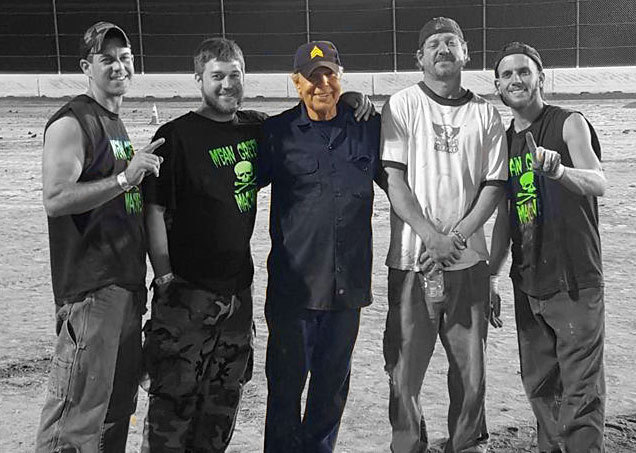/* styles */ Santa Fe Speedway racer, U.S. Army Veteran and father to one of modern day Team Demolition Derby's most recognizable families, Mel Van Allen passed away this week after a lengthy battle with cancer. Mel was a constant figurehead and leader for Mean Green Machine, guiding the success of sons #16 Zac and #14 Jay along side two more sons, Trey and Josh. If dealing with Demos wasn't enough to handle, he also was a father to daughters Amy and Sarah, and grandfather to 11 of the next generation of Van Allen's. His work ethic, skills and general presence on race day will be missed.
 table div table+table+table+table+table+table+table+table+table+table+table+table+table+table+table+table+table+table+table+table+table+table+table+table+table+table+table+table+table+table+table+table+table+table+table div table{width:100%;padding:0}table div table+table+table+table+table+table+table+table+table+table+table+table+table+table+table+table+table+table+table+table+table+table+table+table+table+table+table+table+table+table+table+table+table+table+table div table table{padding:0;float:left!important;width:48.111%!important}table div table+table+table+table+table+table+table+table+table+table+table+table+table+table+table+table+table+table+table+table+table+table+table+table+table+table+table+table+table+table+table+table+table+table+table div table td{padding-left:13px;padding-right:13px}table div table+table+table+table+table+table+table+table+table+table+table+table+table+table+table+table+table+table+table+table+table+table+table+table+table+table+table+table+table+table+table+table+table+table+table div table table td{padding-left:0;padding-right:20px}table div table+table+table+table+table+table+table+table+table+table+table+table+table+table+table+table+table+table+table+table+table+table+table+table+table+table+table+table+table+table+table+table+table+table+table div table table+table{float:left!important;width:51.889%!important}table div table+table+table+table+table+table+table+table+table+table+table+table+table+table+table+table+table+table+table+table+table+table+table+table+table+table+table+table+table+table+table+table+table+table+table div table table+table td{padding-left:0;padding-right:0}/* styles */
 Like   Tweet